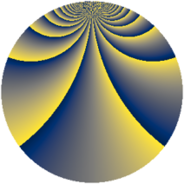# Properties

 Label 1805.2.sLevel $1805$ Weight $2$ Character orbit 1805.s Rep. character $\chi_{1805}(127,\cdot)$ Character field $\Q(\zeta_{36})$ Dimension $1848$ Sturm bound $380$

# Related objects

## Defining parameters

 Level: $$N$$ $$=$$ $$1805 = 5 \cdot 19^{2}$$ Weight: $$k$$ $$=$$ $$2$$ Character orbit: $$[\chi]$$ $$=$$ 1805.s (of order $$36$$ and degree $$12$$) Character conductor: $$\operatorname{cond}(\chi)$$ $$=$$ $$95$$ Character field: $$\Q(\zeta_{36})$$ Sturm bound: $$380$$

## Dimensions

The following table gives the dimensions of various subspaces of $$M_{2}(1805, [\chi])$$.

Total New Old
Modular forms 2520 2232 288
Cusp forms 2040 1848 192
Eisenstein series 480 384 96

## Trace form

 $$1848 q + 12 q^{2} + 12 q^{3} + 12 q^{5} + 12 q^{6} - 6 q^{7} + 18 q^{8} + O(q^{10})$$ $$1848 q + 12 q^{2} + 12 q^{3} + 12 q^{5} + 12 q^{6} - 6 q^{7} + 18 q^{8} + 12 q^{10} + 12 q^{11} + 18 q^{12} + 12 q^{13} - 6 q^{15} - 12 q^{16} + 30 q^{17} - 132 q^{20} - 24 q^{21} + 24 q^{22} - 12 q^{25} + 96 q^{26} + 18 q^{27} + 6 q^{30} + 36 q^{31} - 18 q^{32} - 90 q^{33} + 30 q^{35} - 24 q^{36} - 54 q^{40} - 12 q^{41} - 24 q^{42} - 48 q^{43} - 12 q^{45} + 36 q^{46} + 24 q^{47} - 60 q^{48} - 126 q^{50} + 96 q^{51} + 30 q^{53} - 18 q^{55} - 336 q^{58} - 84 q^{60} + 48 q^{61} - 60 q^{62} + 126 q^{63} - 72 q^{65} - 72 q^{66} - 108 q^{67} - 42 q^{68} - 36 q^{70} + 24 q^{71} - 48 q^{72} - 6 q^{73} - 492 q^{77} + 138 q^{78} + 60 q^{80} + 120 q^{81} - 60 q^{82} - 24 q^{83} + 36 q^{85} + 180 q^{86} + 6 q^{87} + 198 q^{88} + 270 q^{90} + 24 q^{91} + 72 q^{92} + 90 q^{93} - 144 q^{96} + 72 q^{97} + 90 q^{98} + O(q^{100})$$

## Decomposition of $$S_{2}^{\mathrm{new}}(1805, [\chi])$$ into newform subspaces

The newforms in this space have not yet been added to the LMFDB.

## Decomposition of $$S_{2}^{\mathrm{old}}(1805, [\chi])$$ into lower level spaces

$$S_{2}^{\mathrm{old}}(1805, [\chi]) \cong$$ $$S_{2}^{\mathrm{new}}(95, [\chi])$$$$^{\oplus 2}$$